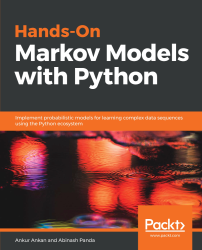•#### Hands-On Markov Models with Python#### Overview of this book

Hidden Markov Model (HMM) is a statistical model based on the Markov chain concept. Hands-On Markov Models with Python helps you get to grips with HMMs and different inference algorithms by working on real-world problems. The hands-on examples explored in the book help you simplify the process flow in machine learning by using Markov model concepts, thereby making it accessible to everyone. Once you’ve covered the basic concepts of Markov chains, you’ll get insights into Markov processes, models, and types with the help of practical examples. After grasping these fundamentals, you’ll move on to learning about the different algorithms used in inferences and applying them in state and parameter inference. In addition to this, you’ll explore the Bayesian approach of inference and learn how to apply it in HMMs. In further chapters, you’ll discover how to use HMMs in time series analysis and natural language processing (NLP) using Python. You’ll also learn to apply HMM to image processing using 2D-HMM to segment images. Finally, you’ll understand how to apply HMM for reinforcement learning (RL) with the help of Q-Learning, and use this technique for single-stock and multi-stock algorithmic trading. By the end of this book, you will have grasped how to build your own Markov and hidden Markov models on complex datasets in order to apply them to projects.
PrefaceFree Chapter
Introduction to the Markov ProcessHidden Markov ModelsState Inference - Predicting the StatesParameter Learning Using Maximum LikelihoodParameter Inference Using the Bayesian ApproachTime Series PredictingNatural Language Processing2D HMM for Image ProcessingMarkov Decision ProcessOther Books You May Enjoy# The HMM

An HMM is a specific case of state space model in which the latent variables are discrete and multinomial variables. From the graphical representation, we can also consider an HMM to be a double stochastic process consisting of a hidden stochastic Markov process (of latent variables) that we cannot observe directly, and another stochastic process that produces a sequence of the observation given the first process.

Before moving on to the parameterization, let's consider an example of coin-tossing to get an idea of how it works. Assume that we have two unfair coins, M1 and M2, with M1 having a higher probability (70%) of getting heads and M2 having a higher probability (80%) of getting tails. Someone sequentially flips these two coins, however, we do not know which one. We can only observe the outcome, which can either be heads (H) or tails (T):We can consider the...